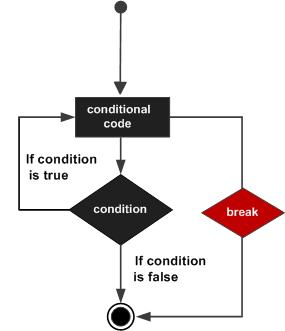## Pascal - Break Statement

Contents[Hide]

The break statement in Pascal has the following two usages:

When the break statement is encountered inside a loop, the loop is immediately terminated and program control resumes at the next statement following the loop.

1. It can be used to terminate a case in the case statement (covered in the next chapter).
2. If you are using nested loops ( ie. one loop inside another loop), the break statement will stop the execution of the innermost loop and start executing the next line of code after the block.

## 1. Syntax:

The syntax for a break statement in Pascal is as follows:

`break;`

## 2. Flow Diagram:## 3. Example:

```program exBreak;
var
a: integer;
begin
a :=10;
while (a<20) do
begin
writeln('value of a: ', a);
a:=a +1;
if (a>15) then
break; {terminate the loop}
end;
end.
```

When the above code is compiled and executed, it produces following result:

```value of a: 10
value of a: 11
value of a: 12
value of a: 13
value of a: 14
value of a: 15

```